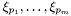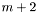#Boost C++ Libraries

...one of the most highly regarded and expertly designed C++ library projects in the world.

This is the documentation for an old version of Boost. Click here to view this page for the latest version.

## Struct template extended_p_square_impl

boost::accumulators::impl::extended_p_square_impl — Multiple quantile estimation with the extendedalgorithm.

## Synopsis

```// In header: <boost/accumulators/statistics/extended_p_square.hpp>

template<typename Sample>
struct extended_p_square_impl {
// types
typedef numeric::functional::average< Sample, std::size_t >::result_type float_type;
typedef std::vector< float_type >                                        array_type;
typedef unspecified                                                      result_type;

// construct/copy/destruct
template<typename Args> extended_p_square_impl(Args const &);

// public member functions
template<typename Args> void operator()(Args const &) ;
result_type result(dont_care) const;
};```

## Description

Extendedalgorithm for estimation of several quantiles without storing samples. Assume thatquantilesare to be estimated. Instead of storing the whole sample cumulative distribution, the algorithm maintains onlyprincipal markers andmiddle markers, whose positions are updated with each sample and whose heights are adjusted (if necessary) using a piecewise-parablic formula. The heights of these central markers are the current estimates of the quantiles and returned as an iterator range.

For further details, see

K. E. E. Raatikainen, Simultaneous estimation of several quantiles, Simulation, Volume 49, Number 4 (October), 1986, p. 159-164.

The extendedalgorithm generalizess thealgorithm of

R. Jain and I. Chlamtac, The P^2 algorithmus for dynamic calculation of quantiles and histograms without storing observations, Communications of the ACM, Volume 28 (October), Number 10, 1985, p. 1076-1085.

### `extended_p_square_impl` public construct/copy/destruct

1. `template<typename Args> extended_p_square_impl(Args const & args);`

### `extended_p_square_impl` public member functions

1. `template<typename Args> void operator()(Args const & args) ;`
2. `result_type result(dont_care) const;`